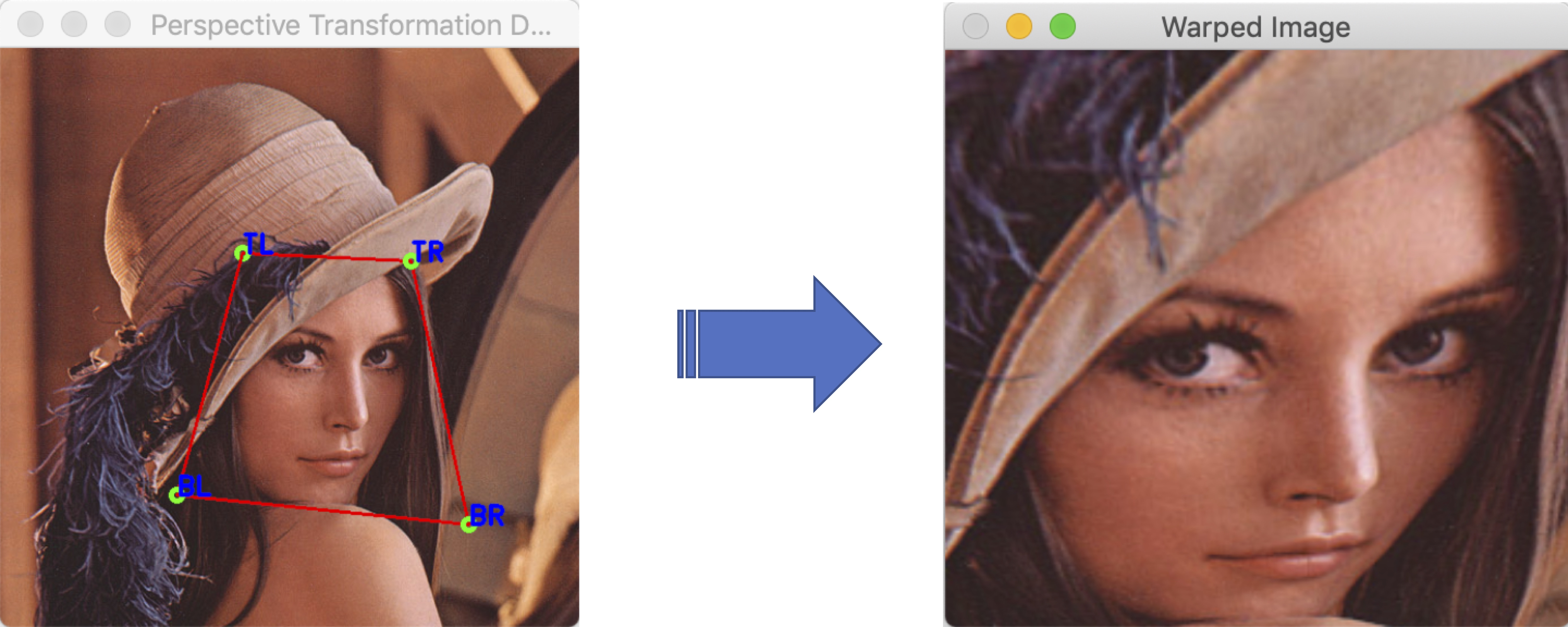# OpenCV C++学习笔记(5): 原位做4点投影变换

OpenCV官方的warpPerspective示例用一个窗口进行选区, 用另一个窗口进行显示:vector<Point2f> d_corner(4);
vector<Point2f> o_corner(4);


void point_warp(
Point2f &p_target,
const Point2f &p,
const Mat &H){
//    映射矩阵H是一个3x3的矩阵,
//    s*[p_target.x, p_target.y, 1]= H * [p.x, p.y, 1]
//    乘出来以后要对第三项归一化
Mat p_vector = (Mat_ <double>(3, 1) << p.x,p.y, 1);
p_vector = H * p_vector;
p_target.x=(float)(p_vector.at<double>(0)/
p_vector.at<double>(2));
p_target.y=(float)(p_vector.at<double>(1)/
p_vector.at<double>(2));
}


• 鼠标在变形图上(x,y)点按下, d_corner[selected_corner_i]=Point2f(x,y);

• 计算出原始图上的对应点位置

point_warp(
o_corner[selected_corner_i],
d_corner[selected_corner_i],
H_inv);

• 鼠标开始拖动, 移动到新的(x,y)位置, 此时新的: d_corner[selected_corner_i]=Point2f(x,y);

• 根据新的o_corner和d_corner分别计算映射矩阵H和逆矩阵H_inv:

H = findHomography(o_corner, d_corner);
H_inv= findHomography(d_corner, o_corner);

• 在根据映射矩阵H, 将原始图映射到变形图上
Mat warped_image;
warpPerspective(img, warped_image, H, Size(width,height));